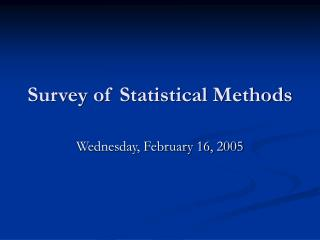Download PresentationSurvey of Statistical Methods

# Survey of Statistical Methods - PowerPoint PPT PresentationDownload Presentation## Survey of Statistical Methods

- - - - - - - - - - - - - - - - - - - - - - - - - - - E N D - - - - - - - - - - - - - - - - - - - - - - - - - - -
##### Presentation Transcript

1. Survey of Statistical Methods Wednesday, February 16, 2005

2. Cereal Box Problem • A cereal company is offering six different prizes in its cereal boxes. • On average, how many boxes would one expect to have to buy in order to collect all six prizes? • If you bought 15 boxes of cereal, but still did not get all six prizes do you have a right to complain to the manufacturer? If so, how would you justify your complaint? • Suppose you suspected that one prize was in shorter supply than the others, how could you design a study to test your hypothesis?

3. Q1: On average, how many boxes would one expect to have to buy in order to collect all six prizes? • Need to create a “probability distribution” using the data that we have collected… • How would you report the “average”?

4. If you bought 15 boxes of cereal, but still did not get all six prizes do you have a right to complain to the manufacturer? If so, how would you justify your complaint? • Probability Theory • Probability theory is the branch of mathematics which develops models for "chance variations" or "random phenomena." It originated as a rigorous discipline when mathematicians of the 17th century began calculating the odds in various games of chance.

5. Suppose you suspected that one prize was in shorter supply than the others, how could you design a study to test your hypothesis? • Statistics • Statistics is the mathematical science of utilizing data about a population in order to describe it usefully and to draw conclusions and make decisions. The population may be a community, an organization, a production line, a service counter, or a phenomenon such as the weather. Statisticians develop models based on probability theory. They determine which probability model is correct for a given type of problem and they decide what kinds of data should be collected and examined.

6. Assignment for Friday • Complete Questions 4.1 through 4.12 (pages 127-130) in your textbook as practice for lab on Friday. • Complete a UTOS worksheet for a research article that you have had to read for another class.

7. Date: 02/06/97 at 21:41:26 • From: Doctor Wallace • Subject: Re: Odds and probability • No, odds is not slang for probability. There is a difference. A probability is a number from 0 to 1 inclusive, usually expressed as a fraction, which is the ratio of the number of chances of a specific event to the total number of chances possible. For example, if I have 4 marbles in a jar, 3 red and 1 blue, then the probability of drawing the blue is 1/4. There is one chance of a blue marble and 4 total chances (marbles). • Odds are expressed as the number of chances for (or against) versus the number of chances against (or for). So, since there is 1 chance of your picking the blue, and 3 chances of your picking red, the odds are 3 to 1 AGAINST you picking the blue. For odds in favor, we just reverse them. The odds are 1 to 3 IN FAVOR OF you picking the blue. • This can be a little confusing, so I'll say it again. If you express odds as AGAINST, you put the number of chances against first, versus the number of chances for. If you express odds as IN FAVOR OF, you put the chances for the event happening first. Note that this does NOT mean that the probability is 1/3 for or against in the above example. To convert odds to probability, we have to ADD the chances. So, if the odds against a horse winning are 4 to 1, this means that, out of 5 (4 + 1) chances, the horse has 1 chance of winning. So the PROBABILITY of the horse winning is 1/5 or 20 percent. • I hope this helps. If you need more assistance, don't hesitate to write back! • -Doctor Wallace, The Math Forum • Check out our web site! http://forum.swarthmore.edu/dr.math/